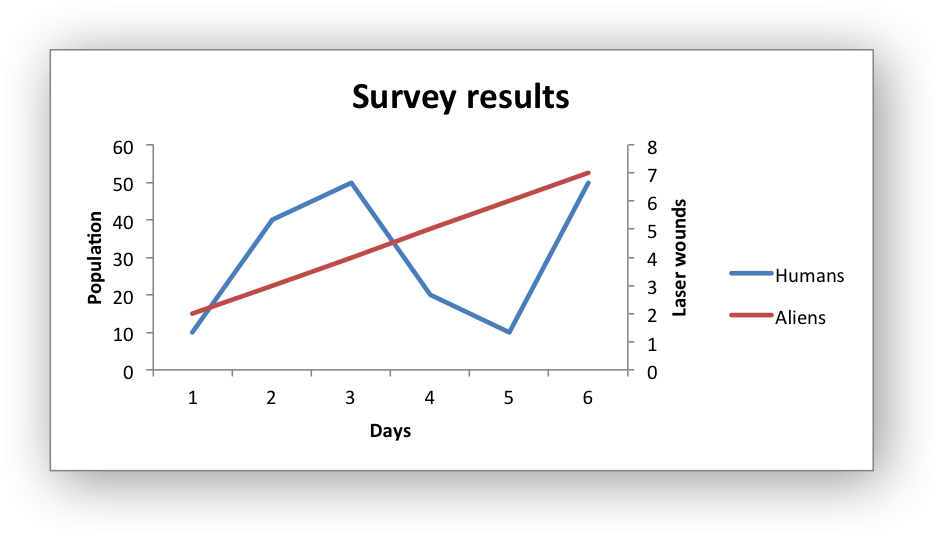# Example: Secondary Axis Chart

Example of creating an Excel Line chart with a secondary axis. Note, the primary and secondary chart type are the same. The next example shows a secondary chart of a different type.```#######################################################################
#
# An example of creating an Excel Line chart with a secondary axis
# using Python and XlsxWriter.
#
# Copyright 2013-2023, John McNamara, jmcnamara@cpan.org
#
import xlsxwriter

workbook = xlsxwriter.Workbook("chart_secondary_axis.xlsx")

# Add the worksheet data that the charts will refer to.
data = [
[2, 3, 4, 5, 6, 7],
[10, 40, 50, 20, 10, 50],
]

worksheet.write_column("A2", data)
worksheet.write_column("B2", data)

# Create a new chart object. In this case an embedded chart.

# Configure a series with a secondary axis
{
"name": "=Sheet1!\$A\$1",
"values": "=Sheet1!\$A\$2:\$A\$7",
"y2_axis": 1,
}
)

{
"name": "=Sheet1!\$B\$1",
"values": "=Sheet1!\$B\$2:\$B\$7",
}
)

chart.set_legend({"position": "right"})

# Add a chart title and some axis labels.
chart.set_title({"name": "Survey results"})
chart.set_x_axis(
{
"name": "Days",
}
)
chart.set_y_axis({"name": "Population", "major_gridlines": {"visible": 0}})
chart.set_y2_axis({"name": "Laser wounds"})

# Insert the chart into the worksheet (with an offset).
worksheet.insert_chart("D2", chart, {"x_offset": 25, "y_offset": 10})

workbook.close()
```